UIHistories Project: A History of the University of Illinois by Kalev Leetaru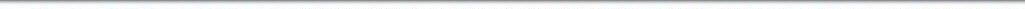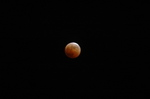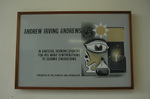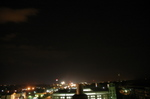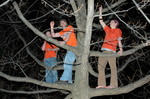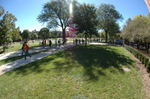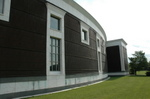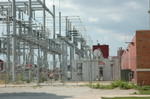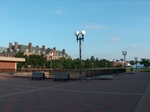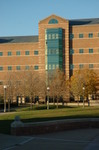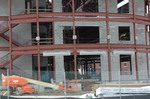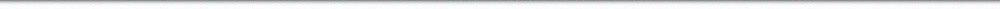N A V I G A T I O N D I G I T A L L I B R A R Y

## Repository: UIHistories Project: Board of Trustees Minutes - 1896 [PAGE 75]

Caption: Board of Trustees Minutes - 1896
This is a reduced-resolution page image for fast online browsing.

< Previous Page [Displaying Page 75 of 371] Next Page >
[VIEW ALL PAGE THUMBNAILS]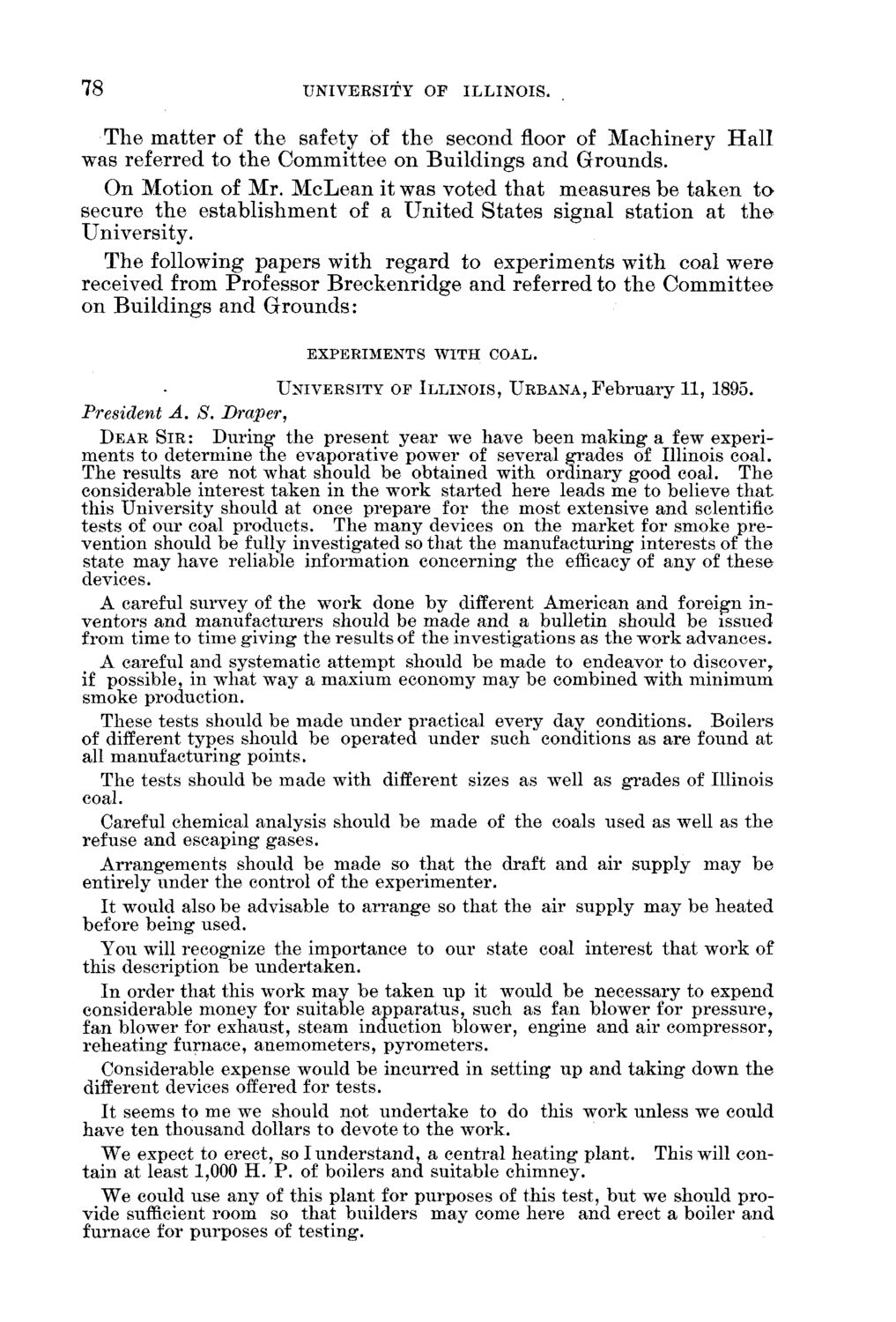### EXTRACTED TEXT FROM PAGE:

78

UNIVERSITY OF

ILLINOIS.

T h e m a t t e r of t h e safety of t h e second floor of M a c h i n e r y H a l l was referred to t h e C o m m i t t e e on B u i l d i n g s a n d G r o u n d s . O n M o t i o n of Mr. M c L e a n it was voted t h a t m e a s u r e s be t a k e n t o secure t h e e s t a b l i s h m e n t of a U n i t e d S t a t e s signal s t a t i o n at t h e University. T h e following p a p e r s w i t h r e g a r d to e x p e r i m e n t s w i t h coal w e r e received from Professor B r e c k e n r i d g e a n d referred to t h e C o m m i t t e e on B u i l d i n g s a n d G r o u n d s :

EXPERIMENTS WITH COAL. UNIVERSITY OF ILLINOIS, U R B ANA, February 11, 1895.